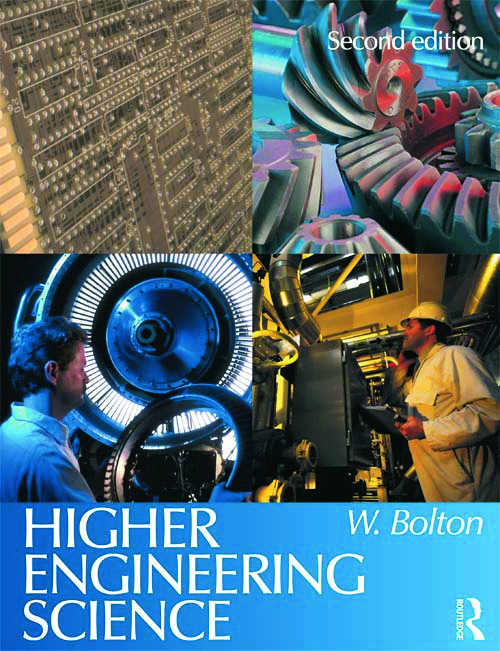# Higher Engineering Science

## 2nd Edition

Routledge

320 pages

Paperback: 9780750662536
pub: 2004-02-10
\$52.95
x
Hardback: 9781138131743
pub: 2015-11-26
\$195.00
x
eBook (VitalSource) : 9780080940199
pub: 2012-08-21
from \$26.48

FREE Standard Shipping!

### Description

Higher Engineering Science aims to provide students with an understanding of the scientific principles that underpin the design and operation of modern engineering systems. It builds a sound scientific foundation for further study of electronics, electrical engineering and mechanical engineering.

The text is ideal for students, including numerous features designed to aid student learning and put theory into practice:
* Worked examples with step-by-step guidance and hints
* Highlighted key points, applications and practical activities
* Self-check questions included throughout the text
* Problems sections with full answers supplied

Further worked examples, applications, case studies and assignments have also been incorporated into this second edition.

Assuming a minimum of prior knowledge, the book has been written to suit courses with an intake from a range of educational backgrounds. The new edition has been designed specifically to cater for the compulsory core Engineering Science unit for HNC and HND qualifications, and updated throughout to match the syllabus of the new BTEC Higher National Engineering schemes from Edexcel. It will also prove ideal for introductory science modules in degree courses.

Structural analysis:
Introduction.
Poisson's ratio.
Problems.

Beams and columns:
Introduction.
Beams.
Shear force and bending moment.
Bending stresses.
Columns.
Problems.

Torsion:
Introduction.
Linear motion.
Two dimensional linear motion.
Angular motion.
Force and linear motion.
Torque and angular motion.
Problems.

Energy transfer:
Introduction.
Work.
Potential energy.
Linear and angular kinetic energy.
Conservation of mechanical energy.
Mechanical power transmission.

Mechanical oscillations:
Introduction.
Simple harmonic motion.
Undamped oscillations.
Damped oscillations.
Forced oscillations.
Problems.

D c theory:
Introduction.
Resistors in d.c. circuits.
Kirchhoff's laws.
Capacitors.
Inductors.
D c motor.
Problems.

A c theory:
Introduction.
Phasors.
Reactance and susceptance.
Phasor relationships for pure components.
Series a c circuits.
Parallel circuits.
Power.
Problems.

Complex waveforms:
Introduction.
The Fourier series.
Circuit analysis with complex waveforms.
Production of harmonics.
Problems.

Transformers:
Introduction.
Basic principles.
Transformer construction.
Impedance matching.
Problems.

Systems:
Introduction.
Basic principles.
Information and signals.
Signal processing.
Measurement systems.
Feedback systems.
Noise.
Problems.

Control systems:
Introduction.
Basic principles.
Electrical switching.
Speed control of motors.
Problems.